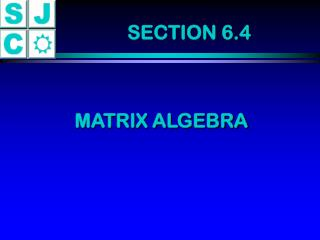DownloadDownload PresentationSECTION 6.4

# SECTION 6.4

Download Presentation## SECTION 6.4

- - - - - - - - - - - - - - - - - - - - - - - - - - - E N D - - - - - - - - - - - - - - - - - - - - - - - - - - -
##### Presentation Transcript

1. SECTION 6.4 MATRIX ALGEBRA

2. THE ALGEBRA OF MATRICES Addition:

3. PROPERTIES OF MATRICES Commutative: A + B = B + A Associative: A + (B + C) = (A + B) + C

4. ZERO FOR MATRICES

6. MORE PROPERTIES OF MATRICES Additive Identity: There is a matrix 0 satisfying 0 + A = A + 0 = A Additive Inverses: For each matrix A, there is a matrix -A satisfying A + (-A) = (-A) + A = 0

7. MORE PROPERTIES OF MATRICES k(A + B) = kA + kB (k + m)A = kA + mA (km)A = k(mA) = m(kA)

8. 3A = -2B = 3A - 2B =

9. MULTIPLICATION: aA+bC aB+bD

10. MULTIPLICATION: aA+bC aB+bD cA+dC cB+dD

11. Find A · B - 19 - 8

12. Find A · B - 19 - 8 17 14

13. Find B · A - 12 23

14. Find B · A - 12 23 2 7

15. MULTIPLICATION: AB = AB ¹ BA Multiplication is not commutative. BA =

16. MORE PROPERTIES OF MATRICES Associative: A · (B · C) = (A · B) · C Distributive: A · (B + C) = A · B + A · C (B + C) · A = B · A + C · A

17. COMPATABILITY OF MATRICES For Addition: Same Size Counterexample:

18. COMPATABILITY OF MATRICES For Multiplication:

19. COMPATABILITY OF MATRICES For Multiplication: Two matrices are compatable for multiplication when the dimensions are: n x m and m x p The product matrix will have dimension n x p

20. USING A CALCULATOR For Multiplication:

21. INVERSES OF MATRICES With matrices, when AB = I = BA, we say A and B are inverses. Furthermore, when a matrix A has an inverse, we denote it as A -1 Many matrices will fail to have an inverse.

22. THEOREM: MULTIPLICATIVE INVERSES The matrix has a multiplicative inverse if and only if D = ad - bc is nonzero.

23. If D ¹ 0, then

24. Does A have an inverse? D = 2(-9) - 5(-5) = -18 + 25 = 7 YES!

25. Does A have an inverse? D = 3(10) - (-6)(-5) = 30 - 30 = 0 NO!

26. INVERSES OF MATRICES This method of finding the inverse of a matrix works nicely on 2 x 2 matrices. Finding an inverse of a 3 x 3 matrix is another matter. We’ll use the calculator!

27. EXAMPLE:

28. APPLICATIONS TO SYSTEMS OF EQUATIONS 2x + 6y + 6z = 2 2x + 7y + 6z = - 3 2x + 7y + 7z = - 5 =

29. APPLICATIONS TO SYSTEMS OF EQUATIONS A- 1 = A- 1 A- 1 =

30. SOLVE THE SYSTEM OF EQUATIONS 2x + 6y + 6z = 2 2x + 7y + 6z = - 3 2x + 7y + 7z = - 5 - 1 =

31. CONCLUSION OF SECTION 6.4